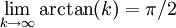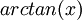# Divergence Test with arctan

Previous: Divergence Test With Square Roots

Next: Video Examples for the Divergence Test

## Question

Use the divergence test to determine whether the infinite seriesdiverges.

## Complete Solution

As always, we apply the divergence theorem by evaluating a limit astends to infinity. In this case we findTherefore, becausedoes not tend to zero as k tends to infinity, the divergence test tells us that the infinite series diverges.

## Potential Challenge Areas

### Remembering What arctan Looks Like

The arctan function is the inverse of the tan function. One way of remembering what it looks like is to remember that the graph of the inverse of a function can be obtained by reflecting it through the straight line y = x. The two functions are shown in the figure below.The graph of arctan(x) (the blue dashed line) can be obtained by reflecting tan(x) (the solid black line) through the line y = x (the dashed black line).

Previous: Divergence Test With Square Roots

Next: Video Examples for the Divergence Test